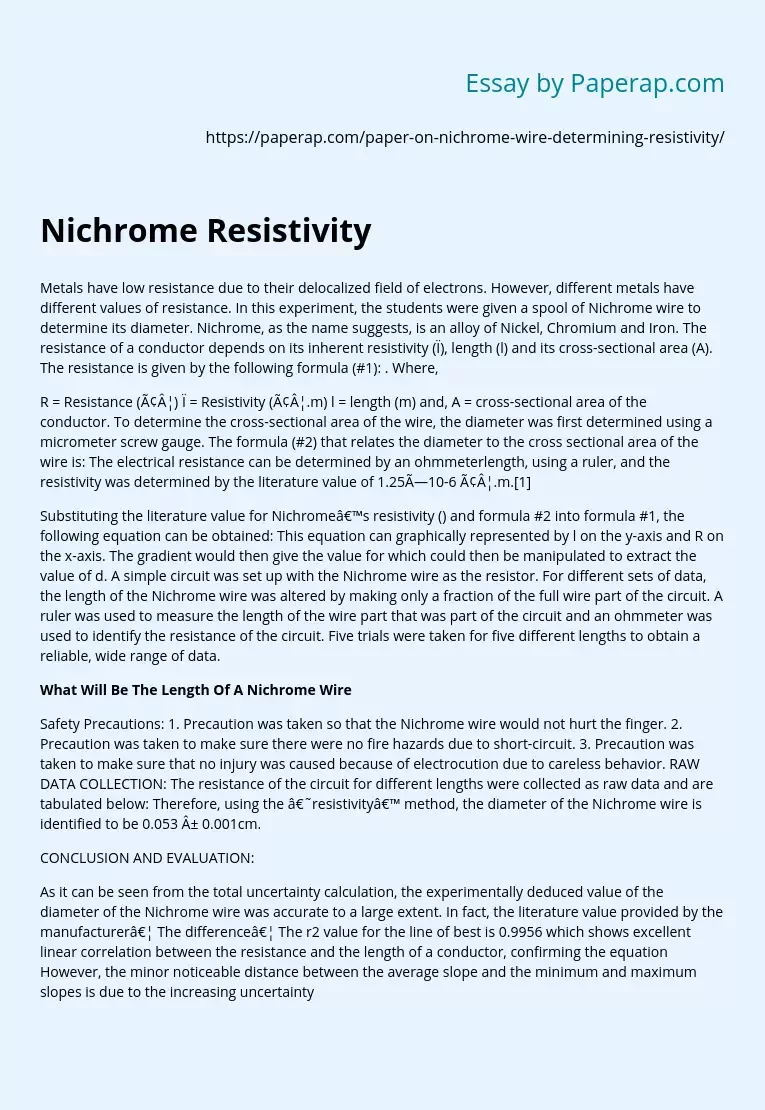# Nichrome Resistivity

Topics: Other

Metals have low resistance due to their delocalized field of electrons. However, different metals have different values of resistance. In this experiment, the students were given a spool of Nichrome wire to determine its diameter. Nichrome, as the name suggests, is an alloy of Nickel, Chromium and Iron. The resistance of a conductor depends on its inherent resistivity (ρ), length (l) and its cross-sectional area (A). The resistance is given by the following formula (#1): . Where,

R = Resistance (â¦) ρ = Resistivity (â¦.m) l = length (m) and, A = cross-sectional area of the conductor.

To determine the cross-sectional area of the wire, the diameter was first determined using a micrometer screw gauge. The formula (#2) that relates the diameter to the cross sectional area of the wire is: The electrical resistance can be determined by an ohmmeterlength, using a ruler, and the resistivity was determined by the literature value of 1.25×10-6 â¦.m.

Substituting the literature value for Nichrome’s resistivity () and formula #2 into formula #1, the following equation can be obtained: This equation can graphically represented by l on the y-axis and R on the x-axis.

The gradient would then give the value for which could then be manipulated to extract the value of d. A simple circuit was set up with the Nichrome wire as the resistor. For different sets of data, the length of the Nichrome wire was altered by making only a fraction of the full wire part of the circuit.

Get quality help nowWriter LylaVerified

Proficient in: Other5 (876)

“ Have been using her for a while and please believe when I tell you, she never fail. Thanks Writer Lyla you are indeed awesome ”+84 relevant experts are online

A ruler was used to measure the length of the wire part that was part of the circuit and an ohmmeter was used to identify the resistance of the circuit. Five trials were taken for five different lengths to obtain a reliable, wide range of data.

## What Will Be The Length Of A Nichrome Wire

Safety Precautions: 1. Precaution was taken so that the Nichrome wire would not hurt the finger. 2. Precaution was taken to make sure there were no fire hazards due to short-circuit. 3. Precaution was taken to make sure that no injury was caused because of electrocution due to careless behavior. RAW DATA COLLECTION: The resistance of the circuit for different lengths were collected as raw data and are tabulated below: Therefore, using the ‘resistivity’ method, the diameter of the Nichrome wire is identified to be 0.053 ± 0.001cm.

CONCLUSION AND EVALUATION:

As it can be seen from the total uncertainty calculation, the experimentally deduced value of the diameter of the Nichrome wire was accurate to a large extent. In fact, the literature value provided by the manufacturer… The difference… The r2 value for the line of best is 0.9956 which shows excellent linear correlation between the resistance and the length of a conductor, confirming the equation However, the minor noticeable distance between the average slope and the minimum and maximum slopes is due to the increasing uncertainty in the measurement of the length.

The uncertainty, however small, cannot be ignored and they must be explored so that if possible, they can be avoided in the future. Firstly, the recording of the length of the Nichrome wire was no so accurate because of the uncertainty in the ruler and its inability to measure all the lengths at one go. A longer ruler or a measuring tape could be used to avoid these kind of easily avoidable errors.

Another problem was that the wire was often crooked at some places and straightening it could have caused it to crack so they were not completely straightened. In fact, the wires were looped around several bobs and the distance between the bobs were measured to give an accurate estimate of the length of the wire. Another issue that arose due to the usage of the bobs was that it was difficult to measure the length of the wire that was used to complete the loop around the bob. A possible workaround both these problems can be to use a thread to run along the Nichrome wire and then measure the thread to determine the length of the Nichrome wire used.

Another limitation to the procedure of the experiment is the assumption of the electrical resistivity of the Nichrome wire. The actual range is 1.00 to 1.50 (×10-6 â¦.m) but the median, 1.25 was assumed. There is no possible way to tell the exact electrical resistivity without knowing the exact composition of the wire and this is because resistivity is a property that is specific to the composition of the conductor and slight changes in the composition can produce significant changes in the value for the resistivity of the conductor.

Depending on the manufacturer’s label for the diameter is accurate but often these values are rounded off to suit the gauge number of the wire. For example, if a 2AWG wire requires a 10mm diameter, most manufacturers will label even a 9.8mm to be 10mm because of their own economic benefits. Therefore, the most accurate way would be use a micrometer screw gauge to measure the diameter of the wire at different locations and then match the experimental value obtained from this experiment against the average of the micrometer readings. This way, dependence on secondary data is minimized and a higher level of confidence can be displayed in the readings.